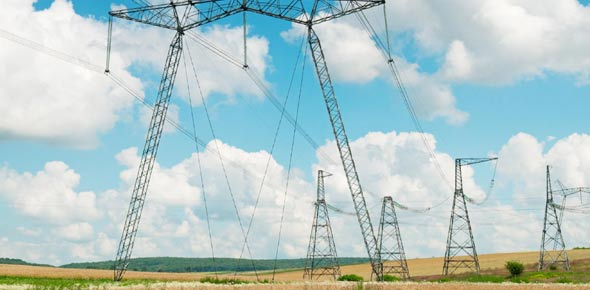# Can You Pass This Hardest Electricity Trivia Quiz?

40 Questions | Total Attempts: 774SettingsCreate your own QuizCheck out our most challenging electricity test and test your knowledge? With this quiz, you will be accountable for understanding what rubber, glass, and dry wood are as far as electricity goes, what are the attributes of a good conductor. How is Ohm’s Law expressed, what occurs when the voltage is doubled, when did the study of electricity first begin, and what is electromotive force. This quiz is all about electricity. You should give it a shot.

• 1.
Concerning the flow of electricity, materials such as rubber, glass, and dry wood are known as
• A.

Conductors

• B.

Insulators

• C.

Thermocouples

• D.

D electric cells

• 2.
Dry wood makes a good conductor.
• A.

True

• B.

False

• 3.
A ______ is the fundamental unit of a battery.
• A.

Watt

• B.

Thermocouple

• C.

Cell

• D.

None of the above answers are correct.

• 4.
Ohm's law may be expressed as an equation I=E/R, where "E" represents:
• A.

Resistance in ohms.

• B.

Voltage in volts

• C.

Current in amperes.

• D.

None of the answers are correct.

• 5.
Ohm's law may be expressed as an equation I=E/R, where "R" represents:
• A.

Resistance in ohms.

• B.

Voltage in volts.

• C.

Current in amperes.

• D.

None of the answers are correct.

• 6.
When the voltage is doubled, power is
• A.

Tripled

• B.

Halved

• C.

Doubled

• D.

Doubled twice

• 7.
The study of electricity began with the ancient___________
• A.

Egyptians

• B.

Jews

• C.

Greeks

• D.

Europeans

• 8.
Charged atoms are called_________
• A.

Ohms

• B.

Conductors

• C.

Photoelectrons

• D.

Ions

• 9.
The flow of electrical energy through a conductor is called
• A.

an electric charge

• B.

Electric current, or electricity.

• C.

a negative charge.

• D.

a unit of conductance.

• 10.
Material that permits the free movement of a large number of electrons, such as copper wire, is known as a good
• A.

Conductor of electricity.

• B.

Insulator of electricity.

• C.

Example of electrolytic action.

• D.

Electromotive force.

• 11.
N order, the best conductors of electricity are
• A.

copper, aluminum, and silver wire.

• B.

aluminum, copper and silver wire.

• C.

Copper, silver, and aluminum wire.

• D.

Silver, copper, and aluminum wire.

• 12.
Electromotive force may be produced by
• A.

Friction and pressure.

• B.

Heat and light.

• C.

Chemical action and magnetism.

• D.

All of the answers are correct.

• 13.
The true cause of electricity was found with the development of
• A.

Charged particles.

• B.

Atomic theory of matter.

• C.

Static electricity.

• D.

Protons.

• 14.
A substance that has few free electrons is called
• A.

Ionic.

• B.

An insulator.

• C.

A conductor.

• D.

Electron is deficient.

• 15.
A common name for the voltage produced by rubbing two materials together is
• A.

Static electricity.

• B.

Friction.

• C.

Dynamic charge

• D.

Dry charge. Dry charge.

• 16.
Wires in an electric circuit are designed to keep
• A.

electrical resistance high.

• B.

Electrical current low.

• C.

electrical resistance low.

• D.

Distance from source voltage to load as far as possible.

• 17.
A significant feature of a battery composed of secondary cells such as an automobile battery is that it is
• A.

cheap.

• B.

Light.

• C.

Rechargeable.

• D.

None of the answers are correct.

• 18.
The direction of electron movement in any conductor is
• A.

From negative potential to positive potential.

• B.

From positive potential to negative potential.

• C.

between like charges.

• D.

in orbit around their nuclei.

• 19.
Which of the following kinds of waves can be felt by human beings?
• A.

Vibrations

• B.

• C.

• D.

Light

• 20.
The ___________ is the length of a cycle expressed in distance units.
• A.

Frequency

• B.

Amplitude

• C.

Wave length

• D.

Cycle

• 21.
The __________ is the number of cycles repeated during one second of time.
• A.

Frequency

• B.

Amplitude

• C.

Speed

• D.

Cycle

• 22.
The __________ is the wave strength at particular points along with the wave.
• A.

Frequency

• B.

Amplitude

• C.

Wave length

• D.

Cycle

• 23.
________occurs when there is a change in the density or atmosphere in which the wave is
• A.

Trapping

• B.

Diffraction

• C.

Refraction

• D.

Reflection

• 24.
_________ causes the spreading of radio waves behind obstructions.
• A.

Trapping

• B.

Diffraction

• C.

Refraction

• D.

Reflection

• 25.
_________ causes the spreading of radio waves behind obstructions.
• A.

Trapping

• B.

Diffraction

• C.

Refraction

• D.

Reflection

Related TopicsBack to top
×

Wait!
Here's an interesting quiz for you.